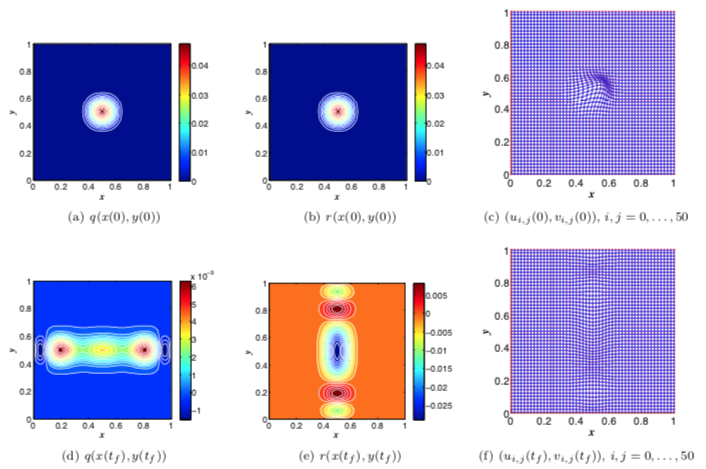# Structure-preserving model reduction for marginally stable LTI systems2D mass–spring example. Initial condition and final state, where the proposed method incurs neglible errors.

### Abstract

This work proposes a structure-preserving model reduction method for marginally stable linear time-invariant (LTI) systems. In contrast to Lyapunov-stability-based approaches—which ensure the poles of the reduced system remain in the open left-half plane—the proposed method preserves marginal stability by reducing the subsystem with poles on the imaginary axis in a manner that ensures those poles remain purely imaginary. In particular, the proposed method decomposes a marginally stable LTI system into (1) an asymptotically stable subsystem with eigenvalues in the open left-half plane and (2) a pure marginally stable subsystem with a purely imaginary spectrum. We propose a method based on inner-product projection and the Lyapunov inequality to reduce the first subsystem while preserving asymptotic stability. In addition, we demonstrate that the pure marginally stable subsystem is a generalized Hamiltonian system; we then propose a method based on symplectic projection to reduce this subsystem while preserving pure marginal stability. In addition, we propose both inner-product and symplectic balancing methods that balance the operators associated with two quadratic energy functionals while preserving asymptotic and pure marginal stability, respectively. We formulate a geometric perspective that enables a unified comparison of the proposed inner-product and symplectic projection methods. Numerical examples illustrate the ability of the method to reduce the dimensionality of marginally stable LTI systems while retaining accuracy and preserving marginal stability; further, the resulting reduced-order model yields a finite infinite-time energy, which arises from the pure marginally stable subsystem.

Publication
Preprint